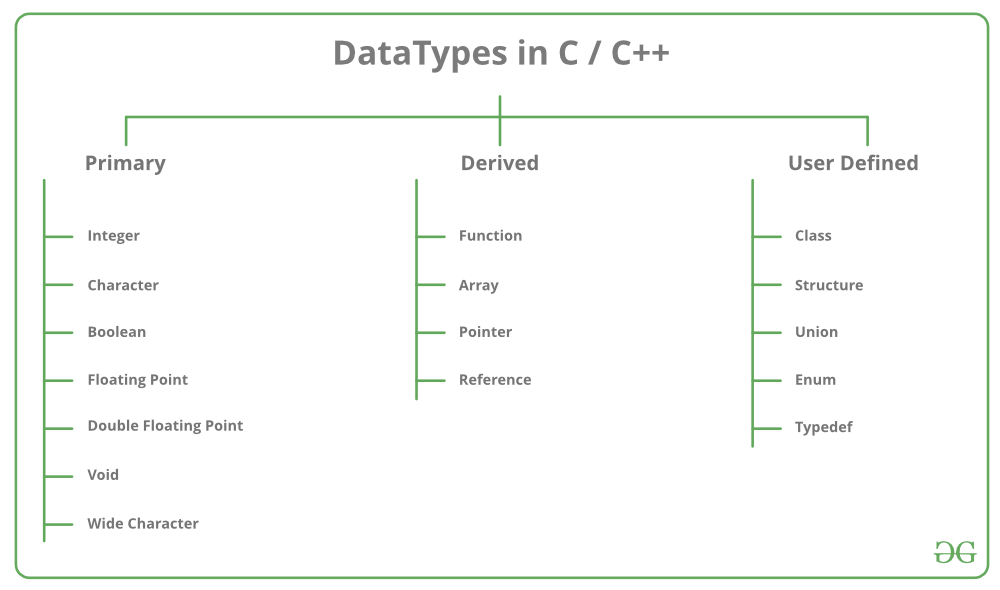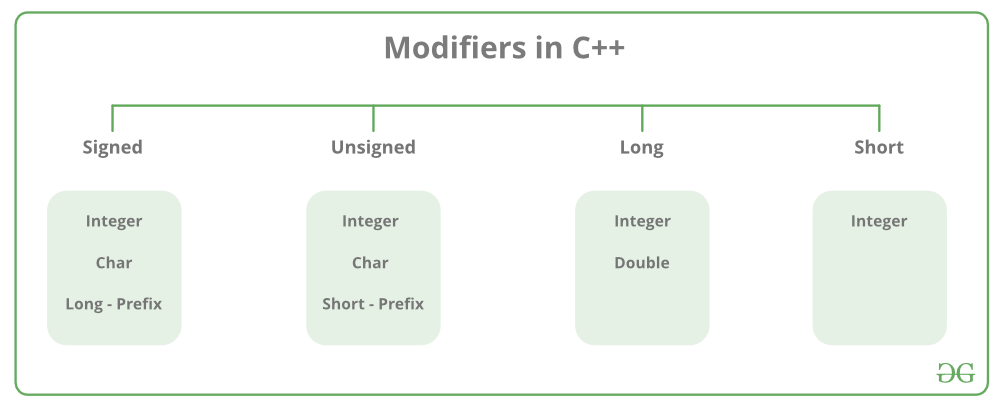Related Articles

# C++ Data Types

• Difficulty Level : Basic
• Last Updated : 21 Apr, 2021

All variables use data-type during declaration to restrict the type of data to be stored. Therefore, we can say that data types are used to tell the variables the type of data it can store. Whenever a variable is defined in C++, the compiler allocates some memory for that variable based on the data-type with which it is declared. Every data type requires a different amount of memory.Data types in C++ is mainly divided into three types:

1. Primitive Data Types: These data types are built-in or predefined data types and can be used directly by the user to declare variables. example: int, char , float, bool etc. Primitive data types available in C++ are:
• Integer
• Character
• Boolean
• Floating Point
• Double Floating Point
• Valueless or Void
• Wide Character
2. Derived Data Types: The data-types that are derived from the primitive or built-in datatypes are referred to as Derived Data Types. These can be of four types namely:
• Function
• Array
• Pointer
• Reference
3. Abstract or User-Defined Data Types: These data types are defined by user itself. Like, defining a class in C++ or a structure. C++ provides the following user-defined datatypes:
• Class
• Structure
• Union
• Enumeration
• Typedef defined DataType

• Integer: Keyword used for integer data types is int. Integers typically requires 4 bytes of memory space and ranges from -2147483648 to 2147483647.

• Character: Character data type is used for storing characters. Keyword used for character data type is char. Characters typically requires 1 byte of memory space and ranges from -128 to 127 or 0 to 255.

• Boolean: Boolean data type is used for storing boolean or logical values. A boolean variable can store either true or false. Keyword used for boolean data type is bool

• Floating Point: Floating Point data type is used for storing single precision floating point values or decimal values. Keyword used for floating point data type is float. Float variables typically requires 4 byte of memory space.

• Double Floating Point: Double Floating Point data type is used for storing double precision floating point values or decimal values. Keyword used for double floating point data type is double. Double variables typically requires 8 byte of memory space.

• void: Void means without any value. void datatype represents a valueless entity. Void data type is used for those function which does not returns a value.

• Wide Character: Wide character data type is also a character data type but this data type has size greater than the normal 8-bit datatype. Represented by wchar_t. It is generally 2 or 4 bytes long.

Datatype Modifiers

As the name implies, datatype modifiers are used with the built-in data types to modify the length of data that a particular data type can hold.Data type modifiers available in C++ are:

• Signed
• Unsigned
• Short
• Long

Below table summarizes the modified size and range of built-in datatypes when combined with the type modifiers:

Note : Above values may vary from compiler to compiler. In the above example, we have considered GCC 32 bit.
We can display the size of all the data types by using the sizeof() operator and passing the keyword of the datatype as argument to this function as shown below:

## CPP

 `// C++ program to sizes of data types``#include``using` `namespace` `std;` `int` `main()``{``    ``cout << ``"Size of char : "` `<< ``sizeof``(``char``)``      ``<< ``" byte"` `<< endl;``    ``cout << ``"Size of int : "` `<< ``sizeof``(``int``)``      ``<< ``" bytes"` `<< endl;``    ``cout << ``"Size of short int : "` `<< ``sizeof``(``short` `int``)``      ``<< ``" bytes"` `<< endl;``    ``cout << ``"Size of long int : "` `<< ``sizeof``(``long` `int``)``       ``<< ``" bytes"` `<< endl;``    ``cout << ``"Size of signed long int : "` `<< ``sizeof``(``signed` `long` `int``)``       ``<< ``" bytes"` `<< endl;``    ``cout << ``"Size of unsigned long int : "` `<< ``sizeof``(unsigned ``long` `int``)``       ``<< ``" bytes"` `<< endl;``    ``cout << ``"Size of float : "` `<< ``sizeof``(``float``)``       ``<< ``" bytes"` `<

Output:

```Size of char : 1 byte
Size of int : 4 bytes
Size of short int : 2 bytes
Size of long int : 8 bytes
Size of signed long int : 8 bytes
Size of unsigned long int : 8 bytes
Size of float : 4 bytes
Size of double : 8 bytes
Size of wchar_t : 4 bytes```

This article is contributed by Harsh Agarwal. If you like GeeksforGeeks and would like to contribute, you can also write an article using contribute.geeksforgeeks.org or mail your article to contribute@geeksforgeeks.org. See your article appearing on the GeeksforGeeks main page and help other Geeks.